#### How To Do A Two Variable Sensitivity Analysis In Excel?

We can use two variable sensitivity analysis to evaluate the validity of a matrix containing a range of values for two variables related to a goal.

If you were interested in taking out a financial loan, you could use this function to model the possible monthly loan payments based on two variables – the amount of money you want to loan and the interest rate p.a. (per annum).

You first identify the range of the two variables, interest rate and loan value. For the loan amount you are willing to consider loans between 80,000 and 200,000. For the interest rate you will consider 7.8% – 9.0%.

Excel uses this range of values for both variables to generate a data table that lists the monthly payment at each intersection of the two variables. So, if I take this loan of 150,000 at 7.9%, on the table I will go to intersection those loan and interest values, in that cell will be the monthly payment.

In Excel we use the Data Table feature to generate this analysis.

## Steps to create a Data Table/Two Variable Sensitivity Analysis

The steps below will take you through the process using sample data. A quick reference guide is available at the end of this document.

1. Open sample spreadsheet – How To Do A Two Variable Sensitivity Analysis In Excel Sample Data

2. This sheet shows the monthly payment on a loan of 100,000 at 8.4% interest is 8,400.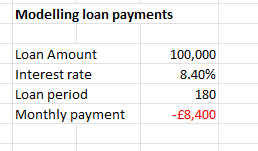3. In cell D9, the PMT function calculates the monthly payment, we will include this equation in the Data Table, where Excel applies this formula to the each of the variables listed.

4. Click in F5, type =D9, press enter. This step is to place the PMT formula within the Data Table.

5. In cell G5, type 80,000, in H5 type 100,000.

6. Select cells G5 and H5, left click the box in the bottom right, drag it to the right until the figure 200,00 displays, release the mouse.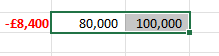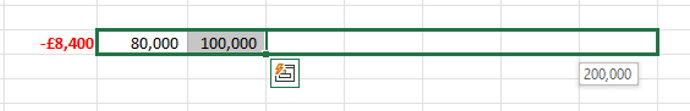7. In cell F6, type 7.8%, in cell F7 type 7.9%.

8. Select both F6 and F7, drag the fill handle down the column to value 9.0%.

9. Select F5 through M18.

10. On the menu click Data, in the Forecast group click What if Analysis, Data Table.

11. The Data Table window opens, the cursor is in the Row input cell. Click D6 as this contains the loan amount which are the values going along the top row of the data table area.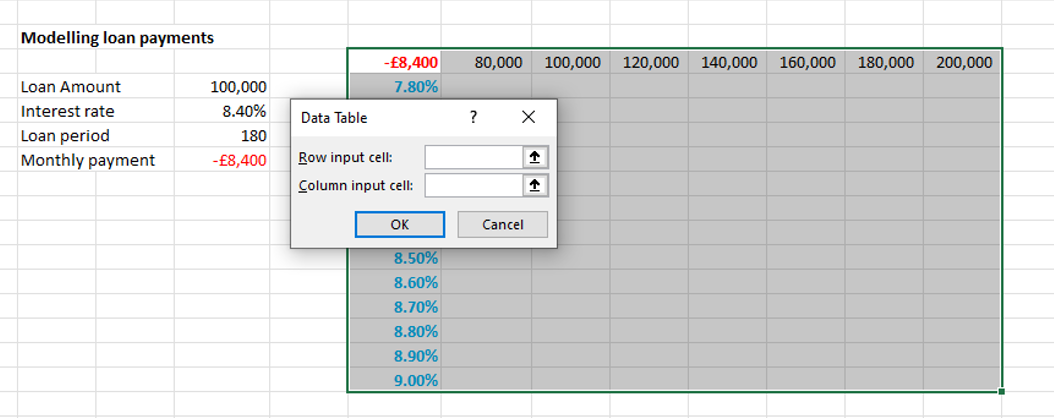12. In the Data Table window, click in the Column input cell field, click D7 to indicate the interest rate is in the first column of the data table.  Click OK.

13. Excel populates the data table with monthly payment amounts. Notice the value at the intersection of 100,000 and 8.4% is correct at 8,400, confirming the results of the PMT equation in D9.

We can now observe the monthly payment in multiple scenarios (e.g., taking out a larger loan at a smaller interest rate) and be confident that the values are valid.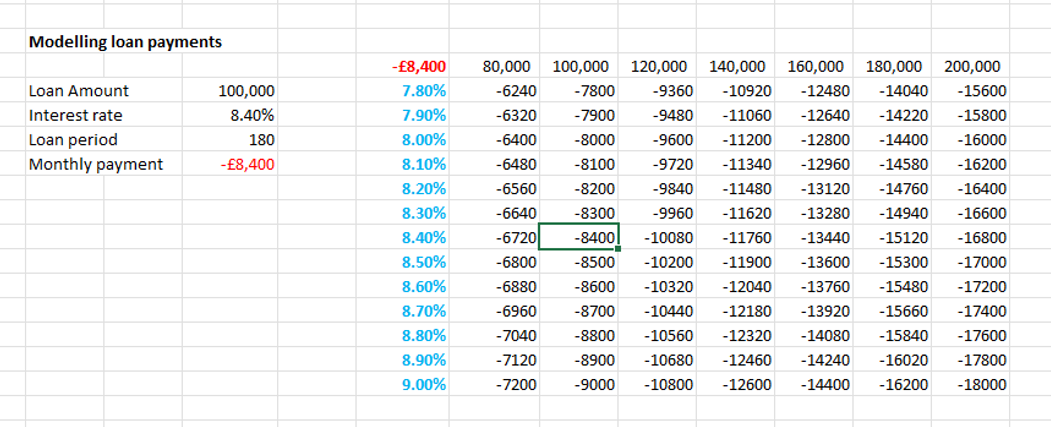The final step in performing a two-variable sensitivity analysis is to interpret the results. This involves analyzing the data table and charts to understand the relationship between X, Y, and Z, and how changes in one variable impact the other.

Interpreting the results of a two-variable sensitivity analysis involves analyzing the data table and charts to understand the relationship between the two variables and how changes in one variable impact the other. It is important to understand the results in order to make informed decisions and determine the relative importance of different variables.

Here are some key steps to help you interpret the results of a two-variable sensitivity analysis:

• Identify trends: Look for patterns in the data table and charts to identify any trends or relationships between the two variables. For example, you may notice that changes in one variable have a greater impact on the outcome than changes in the other variable.
• Evaluate the impact of changes: Analyze the data table to determine the impact of changes in each variable on the outcome. This can help you understand the relative importance of different variables and how they interact with each other.
• Determine the optimal values: Based on the results of the analysis, determine the optimal values for each variable in order to achieve the desired outcome.
• Make informed decisions: Use the results of the analysis to make informed decisions. For example, if you find that changes in one variable have a greater impact on the outcome, you may decide to focus on that variable in order to improve the outcome.

It is important to keep in mind that a two-variable sensitivity analysis is only one tool for evaluating the impact of changes in variables on an outcome. It is important to consider other factors, such as the limitations of the data and model, as well as any assumptions made during the analysis, before making any decisions based on the results.

Overall, interpreting the results of a two-variable sensitivity analysis requires a careful and thorough examination of the data and charts, as well as an understanding of the relationships between the variables and the impact of changes in each variable on the outcome.

For example, if you see that changes in X have a greater impact on Z than changes in Y, you can conclude that X is a more important variable in determining the outcome or result.

## Quick reference guide

1. Open a spreadsheet, list the data and formula you would like to analyse. The two variables and the equation must be in separate cells.  We have created a list of abbreviations to make the steps easier to follow.
• Variable A (row)                       = VA
• Variable B (column)                  = VB
• Equation                                  = E
• Data Table location                  = DTL
2. Identify the cell references for the items in number 1.
3. Identify where you want the Data Table to appear. In the DTL type =E (equation cell reference).
4. In the cell to the right of DTL, type the range of values you want to include in the Data Table row.
5. In the cell under DTL, type the range of values you want to include in the Data Table column.
6. Click the DTL Select all the cells along that row and all the columns to encompass the table and all the variables.
7. On the menu click Data, in the Forecast group click What if Analysis, Data Table.
8. The Data Table window opens, the cursor is in the Row input cell. Click VA, to refer to the values in the top row of the Data Table.
9. In the Data Table window, click in the Column input cell field, click VB to refer to the values in the first column of the Data Table.  Click OK.
10. Excel applies the equation in DTL to all the variables within the table.